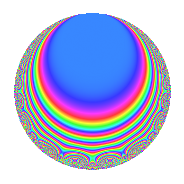# Properties

 Label 51.2.hLevel 51 Weight 2 Character orbit h Rep. character $$\chi_{51}(19,\cdot)$$ Character field $$\Q(\zeta_{8})$$ Dimension 8 Newforms 1 Sturm bound 12 Trace bound 0

# Related objects

## Defining parameters

 Level: $$N$$ = $$51 = 3 \cdot 17$$ Weight: $$k$$ = $$2$$ Character orbit: $$[\chi]$$ = 51.h (of order $$8$$ and degree $$4$$) Character conductor: $$\operatorname{cond}(\chi)$$ = $$17$$ Character field: $$\Q(\zeta_{8})$$ Newforms: $$1$$ Sturm bound: $$12$$ Trace bound: $$0$$

## Dimensions

The following table gives the dimensions of various subspaces of $$M_{2}(51, [\chi])$$.

Total New Old
Modular forms 32 8 24
Cusp forms 16 8 8
Eisenstein series 16 0 16

## Trace form

 $$8q - 8q^{5} - 8q^{6} + O(q^{10})$$ $$8q - 8q^{5} - 8q^{6} + 8q^{11} - 16q^{14} + 16q^{16} - 8q^{17} - 8q^{19} + 16q^{20} - 8q^{22} + 8q^{23} + 8q^{24} - 16q^{25} + 16q^{26} - 8q^{28} + 8q^{31} + 8q^{33} - 8q^{34} + 32q^{35} + 8q^{36} - 8q^{37} + 16q^{39} - 8q^{40} - 24q^{41} + 8q^{42} - 8q^{43} - 8q^{45} - 8q^{49} - 32q^{50} - 16q^{52} - 32q^{53} - 8q^{54} - 16q^{56} - 16q^{57} + 24q^{58} + 16q^{59} - 8q^{60} + 16q^{61} + 16q^{62} + 24q^{65} - 16q^{66} - 16q^{67} - 24q^{69} + 40q^{70} - 16q^{71} + 48q^{73} + 64q^{74} + 16q^{75} + 24q^{76} + 8q^{78} - 16q^{80} - 8q^{82} + 32q^{83} + 40q^{85} - 32q^{86} + 24q^{87} - 8q^{88} - 16q^{91} - 32q^{92} - 32q^{93} - 40q^{95} - 16q^{96} + 8q^{97} + 8q^{99} + O(q^{100})$$

## Decomposition of $$S_{2}^{\mathrm{new}}(51, [\chi])$$ into irreducible Hecke orbits

Label Dim. $$A$$ Field CM Traces $q$-expansion
$$a_2$$ $$a_3$$ $$a_5$$ $$a_7$$
51.2.h.a $$8$$ $$0.407$$ $$\Q(\zeta_{16})$$ None $$0$$ $$0$$ $$-8$$ $$0$$ $$q+(\zeta_{16}+\zeta_{16}^{3})q^{2}+\zeta_{16}^{7}q^{3}+(\zeta_{16}^{2}+\cdots)q^{4}+\cdots$$

## Decomposition of $$S_{2}^{\mathrm{old}}(51, [\chi])$$ into lower level spaces

$$S_{2}^{\mathrm{old}}(51, [\chi]) \cong$$ $$S_{2}^{\mathrm{new}}(17, [\chi])$$$$^{\oplus 2}$$